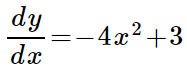### Day 49 - Antiderivative - 10.27.14

 UpdatesGo over Applications of Differentiation TestBell RingerAntiderivativeWhat is the function that has a derivative of f’(x) = 2?f(x) = 2xf(x) = x2f(x) = 2x + 1f(x) = 2x - 3more than one answer existsWhich of the following functions have a derivative of f’(x) = 7x and f(0) = 7f(x) = x2 + 7f(x) = (7/2)x2 + 8f(x) = (7/2)x2 + 7f(x) = -(7/2)x2 - 7none of the aboveWhich of the following functions have a second derivative of f”(x) = 5f(x) = 5xf(x) = (5/2)x2 + 4f(x) = (5/2)x2 - 4more than one answer existsnone of the aboveSolve for y:y = (-4/3)x3 + 3x + Cy = (4/3)x3 + 3x + Cy = (-4/3)x3 - 3x + Cy = (4/3)x3 - 3x + Cnone of the aboveReviewPrerequisitesDerivativesDerivative RulesPower RuleConstant RuleTrig DerivativesLessonIf the derivative of a function is f'(x) = 3, what is the function?AntiderivativeIndefinite IntegralDefinitionIntegral SignIntegrandVariable of IntegrationConstant of IntegrationGeneral SolutionBasic Integration RulesCheckpointsExit TicketPosted on the board at end of the block. Lesson Objective(s)How can indefinite integrals be evaluated using basic integration rules?How are antiderivatives related to derivatives?Standard(s)APC.10Use Riemann sums and the Trapezoidal Rule to approximate definite integrals of functions represented algebraically, graphically, and by a table of values and will interpret the definite integral as the accumulated rate of change of a quantity over an interval interpreted as the change of the quantity over the intervalRiemann sums will use left, right, and midpoint evaluation points over equal subdivisions.APC.11​The student will find antiderivatives directly from derivatives of basic functions and by substitution of variables (including change of limits for definite integrals).APC.12​The student will identify the properties of the definite integral. This will include additivity and linearity, the definite integral as an area, and the definite integral as a limit of a sum as well as the fundamental theorem.APC.13​The student will use the Fundamental Theorem of Calculus to evaluate definite integrals, represent a particular antiderivative, and facilitate the analytical and graphical analysis of functions so defined.APC.14​The student will find specific antiderivatives, using initial conditions (including applications to motion along a line). Separable differential equations will be solved and used in modeling (in particular, the equation y' = ky and exponential growth).APC.15​The student will use integration techniques and appropriate integrals to model physical, biological, and economic situations. The emphasis will be on using the integral of a rate of change to give accumulated change or on using the method of setting up an approximating Riemann sum and representing its limit as a definite integral. Specific applications will includea)​ the area of a region;b)​ the volume of a solid with known cross-section;c)​ the average value of a function; andd) ​the distance traveled by a particle along a line.Mathematical Practice(s)#1 - Make sense of problems and persevere in solving them#2 - Reason abstractly and quantitatively#5 - Use appropriate tools strategically#6 - Attend to precision#8 - Look for and express regularity in repeated reasoningPast CheckpointsN/A